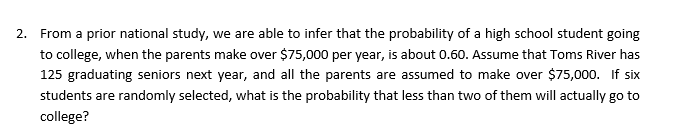# From a prior national study, we are able to infer that the probability of a high school student goingto college, when the parents make over \$75,000 per year, is about 0.60. Assume that Toms River has125 graduating seniors next year, and all the parents are assumed to make over \$75,000. If sixstudents are randomly selected, what is the probability that less than two of them will actually go tocollege?2.

Question
13 viewshelp_outlineImage TranscriptioncloseFrom a prior national study, we are able to infer that the probability of a high school student going to college, when the parents make over \$75,000 per year, is about 0.60. Assume that Toms River has 125 graduating seniors next year, and all the parents are assumed to make over \$75,000. If six students are randomly selected, what is the probability that less than two of them will actually go to college? 2. fullscreen
check_circle

Step 1

In this question, we have given that probability that a student going to college when parents make over \$75000 is equal to 0.60. We have randomly selected 6 students whose parents make over \$75000 and we have to calculate the probability that less than two will actually go to college.

Step 2

Probability less than 2 means either equal to 0 or 1. So, to get the probability we ...

### Want to see the full answer?

See Solution

#### Want to see this answer and more?

Solutions are written by subject experts who are available 24/7. Questions are typically answered within 1 hour.*

See Solution
*Response times may vary by subject and question.
Tagged in
MathStatistics

### Other